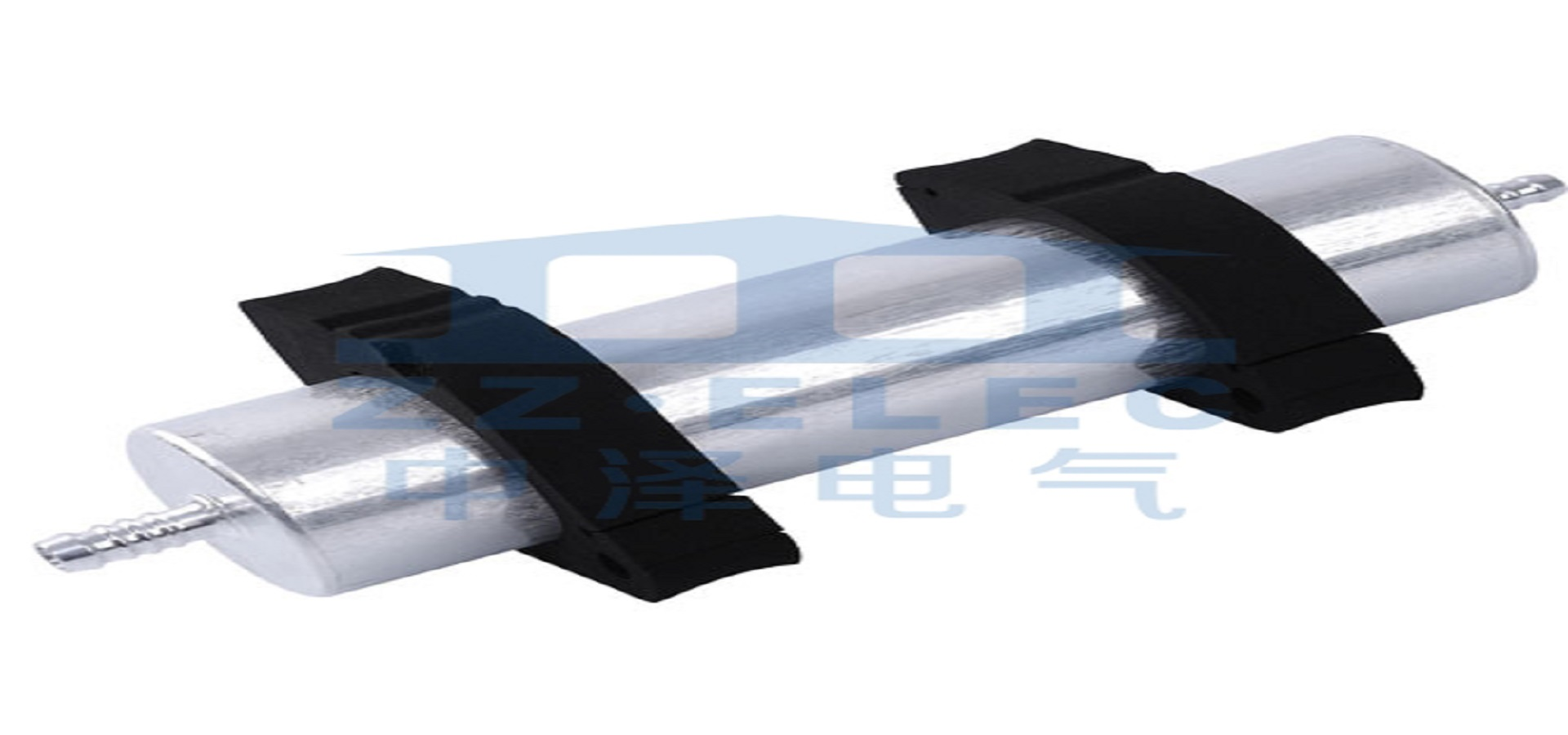# What is the classification and function of capacitors?

Update:21,Mar,2022
Summary:Capacitors are composed of two metal poles with insulating material (dielectric)...
Capacitors are composed of two metal poles with insulating material (dielectric) sandwiched between them. Due to different insulating materials, the types of capacitors formed are also different:
According to the structure, it can be divided into: fixed capacitor, variable capacitor, trimmer capacitor.
According to the dielectric material, it can be divided into: gas dielectric capacitors, liquid dielectric capacitors, inorganic solid dielectric capacitors, organic solid dielectric capacitors, electrolytic capacitors.
According to polarity, it is divided into: polar capacitors and non-polar capacitors. The most common one we see is electrolytic capacitors.
Capacitors have the function of blocking direct current and passing alternating current in the circuit, so they are often used for inter-stage coupling, filtering, decoupling, bypassing and signal tuning. Second, the symbol of capacitance The symbol of capacitance is also divided into domestic standard representation and international electronic symbol representation The only difference is that for polar capacitors, the domestic one is a horizontal line under an empty basket, while the international one is an ordinary capacitor plus a "+" symbol to represent the positive electrode .Unit of capacitance
The basic unit of resistance is: F (method), in addition to μF (microfarad), pF (picofarad), and there is another unit that is used less frequently, that is: nF (), because the capacity of capacitor F is very Therefore, what we see are generally the units of μF, nF, and pF, not the units of F. The specific conversion between them is as follows: 1F=1000000μF1μF=1000nF=1000000pF

Capacitor withstand voltage
Unit: V (volt) Each capacitor has its withstand voltage value, which is one of the important parameters of the capacitor. The nominal withstand voltage values ​​of ordinary non-polar capacitors are: 63V, 100V, 160V, 250V, 400V, 600V, 1000V, etc., the withstand voltage of polar capacitors is relatively lower than that of non-polar capacitors. The general nominal withstand voltage values ​​are: 4V, 6.3V, 10V, 16V, 25V, 35V, 50V, 63V, 80V , 100V, 220V, 400V, etc.